Changing of trignometric variables and then applying formula

Chapter 2 Class 12 Inverse Trigonometric Functions
Concept wise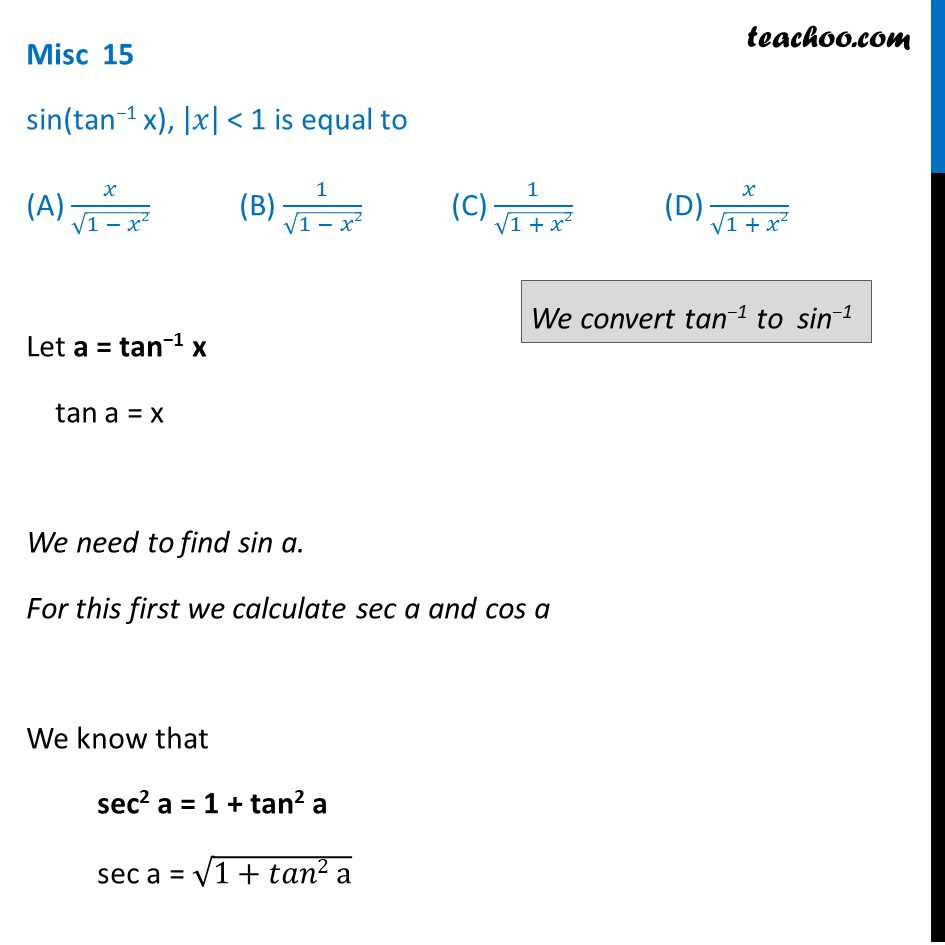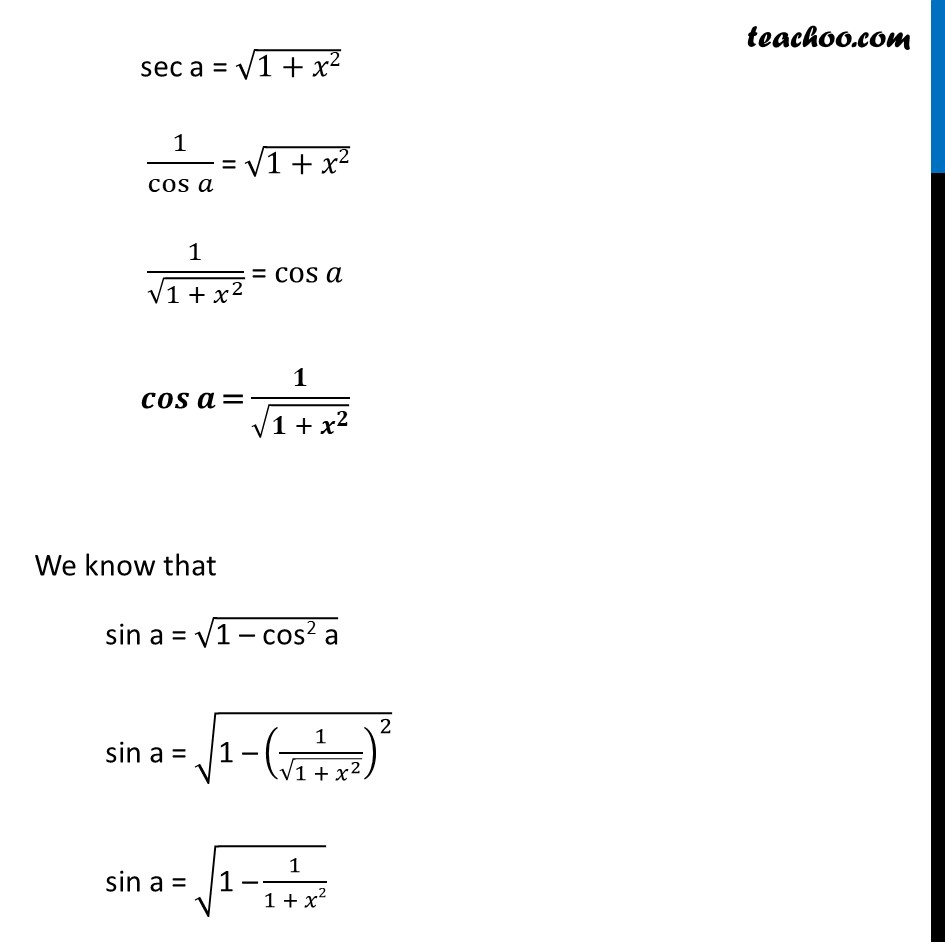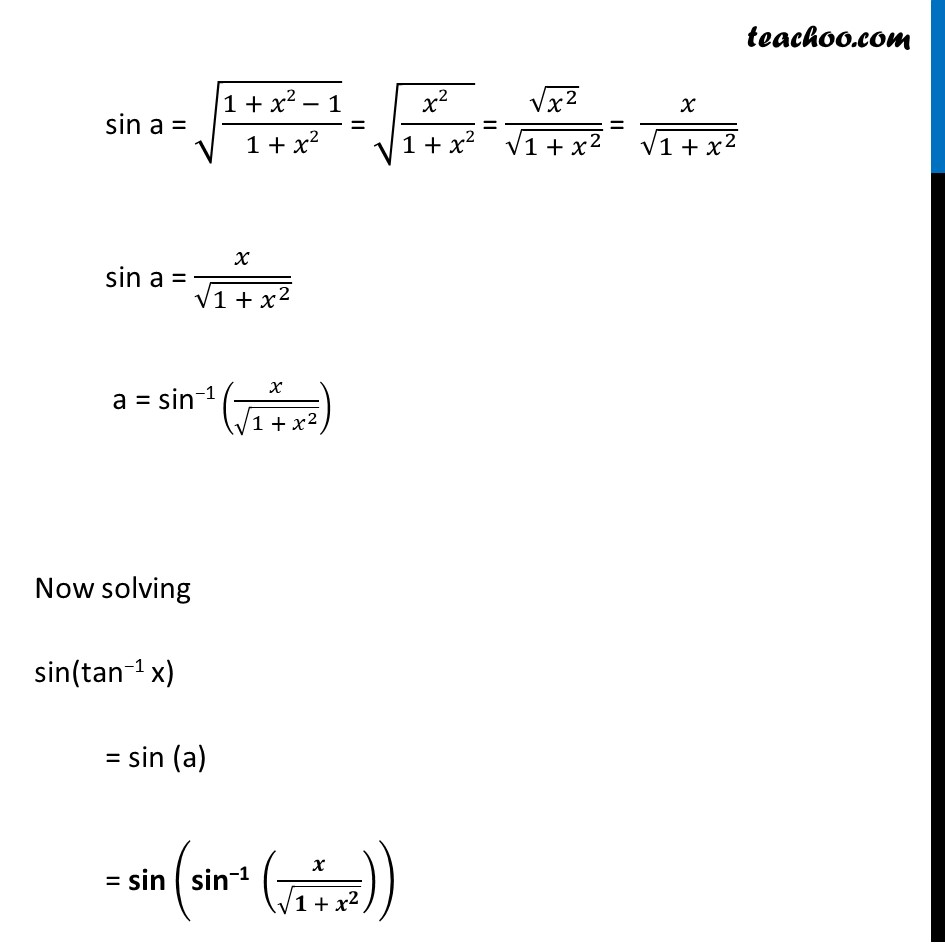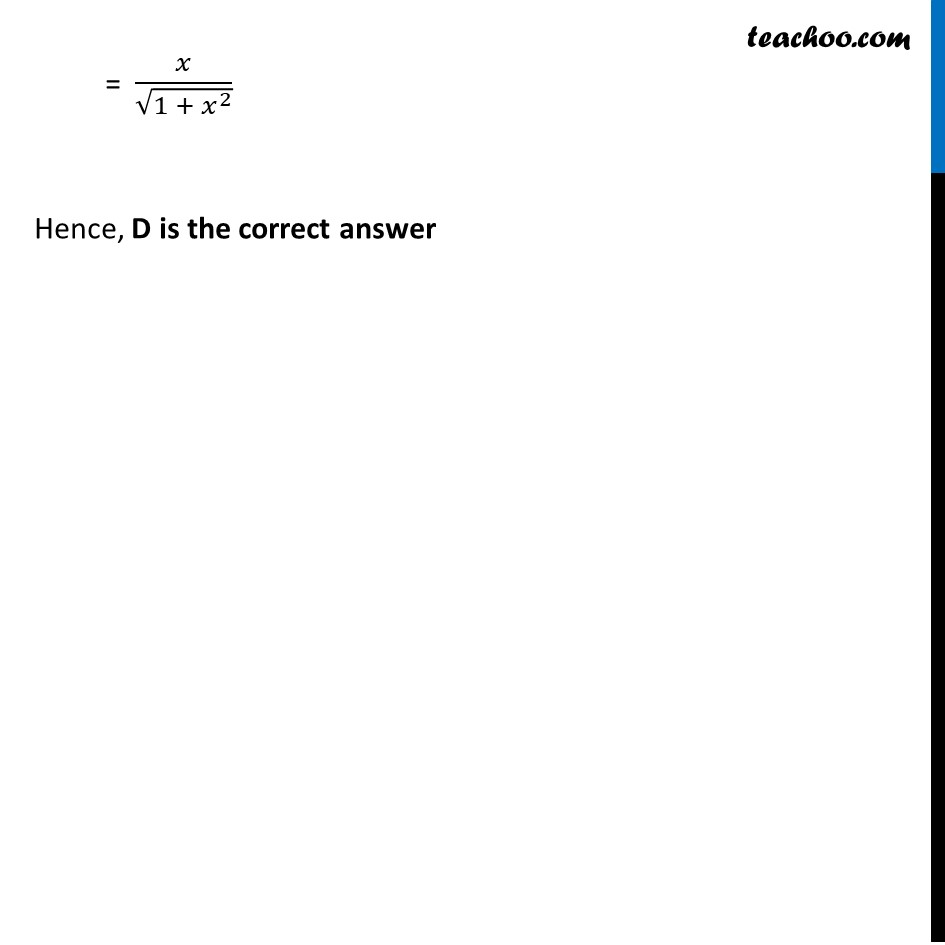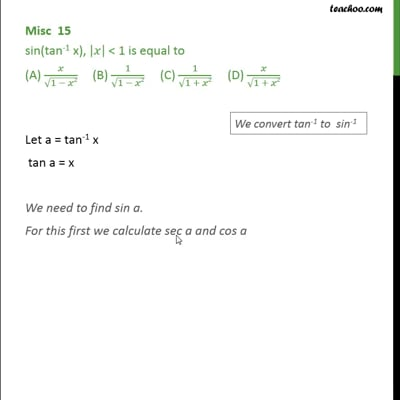This video is only available for Teachoo black users

Introducing your new favourite teacher - Teachoo Black, at only ₹83 per month

### Transcript

Misc 15 sin(tan−1 x), |𝑥| < 1 is equal to (A) 𝑥/√(1 − 𝑥2) (B) 1/√(1 − 𝑥2) (C) 1/√(1 + 𝑥2) (D) 𝑥/√(1 + 𝑥2) Let a = tan−1 x tan a = x We need to find sin a. For this first we calculate sec a and cos a We know that sec2 a = 1 + tan2 a sec a = √(1+𝑡𝑎𝑛2 a) We convert tan−1 to sin−1 sec a = √(1+𝑥2) 1/cos⁡𝑎 = √(1+𝑥2) 1/√(1 + 𝑥^2 ) = cos⁡𝑎 𝒄𝒐𝒔⁡𝒂 = 𝟏/√(𝟏 + 𝒙^𝟐 ) We know that sin a = √("1 – cos2 a" ) sin a = √("1 –" (1/√(1 + 𝑥^2 ))^2 ) sin a = √("1 –" 1/(1 + 𝑥2)) sin a = √((1 + 𝑥2 − 1)/(1 + 𝑥2)) = √((𝑥2 )/(1 + 𝑥2)) = √(𝑥^2 )/√(〖1 + 𝑥〗^2 ) = 𝑥/√(〖1 + 𝑥〗^2 ) sin a = 𝑥/√(〖1 + 𝑥〗^2 ) a = sin−1 (𝑥/√(〖1 + 𝑥〗^2 )) Now solving sin(tan−1 x) = sin (a) = sin ("sin−1 " (𝒙/√(〖𝟏 + 𝒙〗^𝟐 ))) = 𝑥/√(〖1 + 𝑥〗^2 ) Hence, D is the correct answer